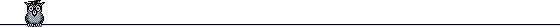# LYAPACK

LYAPACK is a MATLAB toolbox (i.e., a set of MATLAB routines) for the solution of certain large scale problems in control theory, which are closely related to Lyapunov equations. It uses iterative algorithms and is intended for large, sparse problems.

Amongst other things, it can solve Lyapunov and Riccati equations, and do model reduction. Even though LYAPACK has been implemented in MATLAB rather than programming languages like FORTRAN, C, or JAVA, this does not mean that LYAPACK is restricted to the solution of "toy problems". Several measures, such as the use of global variables for large data structures, have been taken to enhance the computational performance of LYAPACK routines. To put this into the right perspective, Lyapunov equations of order larger than 12000 were solved by LYAPACK within few hours on a regular workstation. When using standard methods, supercomputers are needed to solve problems of this size.